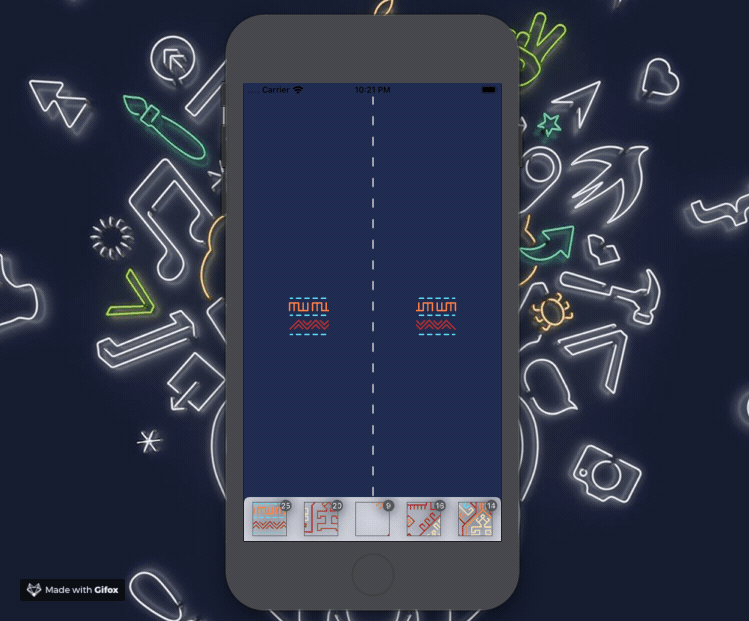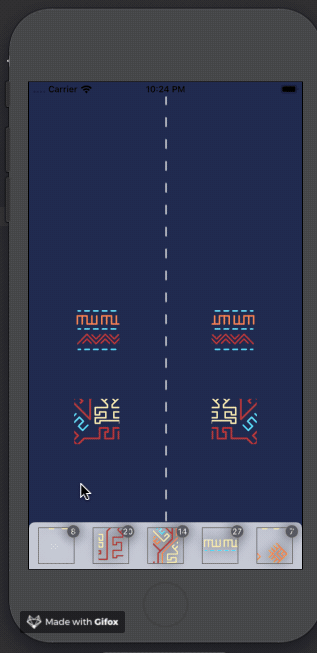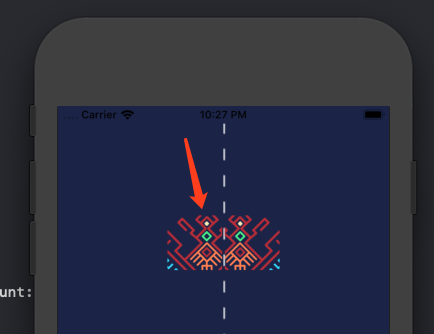Swift 游戏开发之黎锦拼图（四）

## 磁吸效果class ViewController: UIViewController {

// ...

// ...

bottomView.moveBegin = { puzzle in
puzzle.panEnded = {
for copyPuzzle in self.rightPuzzles {
if copyPuzzle.tag == puzzle.tag {
copyPuzzle.copyPuzzleCenterChange(centerPoint: puzzle.center)
}
}
}
// ...
}

bottomView.moveEnd = {
// ...

}
}

/// 启动磁吸
guard let tempPuzzle = self.leftPuzzles.last else { return }

var tempPuzzleCenterPoint = tempPuzzle.center

var tempPuzzleXIndex = CGFloat(Int(tempPuzzleCenterPoint.x / tempPuzzle.width))
if Int(tempPuzzleCenterPoint.x) % Int(tempPuzzle.width) > 0 {
tempPuzzleXIndex += 1
}

var tempPuzzleYIndex = CGFloat(Int(tempPuzzleCenterPoint.y / tempPuzzle.height))
if Int(tempPuzzleCenterPoint.y) % Int(tempPuzzle.height) > 0 {
tempPuzzleYIndex += 1
}

let Xedge = tempPuzzleXIndex * tempPuzzle.width
let Yedge = tempPuzzleYIndex * tempPuzzle.height

if tempPuzzleCenterPoint.x < Xedge {
tempPuzzleCenterPoint.x = Xedge - tempPuzzle.width / 2
}

if tempPuzzleCenterPoint.y < Yedge {
tempPuzzleCenterPoint.y = Yedge  - tempPuzzle.height / 2
}

tempPuzzle.center = tempPuzzleCenterPoint
}

}

## 互斥逻辑

### 拼图 A 和 B 均已在画布上，A 往 B 的位置上移动

class ViewController: UIViewController {

// ...
private var finalPuzzleTags = [[Int]]()

// ...

// 一行六个
let itemHCount = 3
let itemW = Int(view.width / CGFloat(itemHCount * 2))
let itemH = itemW
let itemVCount = Int(contentImageView.height / CGFloat(itemW))

finalPuzzleTags = Array(repeating: Array(repeating: -1, count: itemHCount), count: itemVCount)

// ...
}
}

/// 启动磁吸
private func adsorb(_ tempPuzzle: Puzzle) {
var tempPuzzleCenterPoint = tempPuzzle.center

var tempPuzzleXIndex = CGFloat(Int(tempPuzzleCenterPoint.x / tempPuzzle.width))
if Int(tempPuzzleCenterPoint.x) % Int(tempPuzzle.width) > 0 {
tempPuzzleXIndex += 1
}

var tempPuzzleYIndex = CGFloat(Int(tempPuzzleCenterPoint.y / tempPuzzle.height))
if Int(tempPuzzleCenterPoint.y) % Int(tempPuzzle.height) > 0 {
tempPuzzleYIndex += 1
}

let Xedge = tempPuzzleXIndex * tempPuzzle.width
let Yedge = tempPuzzleYIndex * tempPuzzle.height

if tempPuzzleCenterPoint.x < Xedge {
tempPuzzleCenterPoint.x = Xedge - tempPuzzle.width / 2
}

if tempPuzzleCenterPoint.y < Yedge {
tempPuzzleCenterPoint.y = Yedge  - tempPuzzle.height / 2
}

// 超出最下边
if (Int(tempPuzzleYIndex) > self.finalPuzzleTags.count) {
tempPuzzle.center = tempPuzzle.beginMovedPoint
}

// 已经有的不能占据
if (self.finalPuzzleTags[Int(tempPuzzleYIndex - 1)][Int(tempPuzzleXIndex - 1)] == -1) {
self.finalPuzzleTags[Int(tempPuzzleYIndex - 1)][Int(tempPuzzleXIndex - 1)] = tempPuzzle.tag

if ((tempPuzzle.Xindex != nil) && (tempPuzzle.Yindex != nil)) {
self.finalPuzzleTags[tempPuzzle.Xindex!][tempPuzzle.Yindex!] = -1
}

tempPuzzle.Xindex = Int(tempPuzzleYIndex - 1)
tempPuzzle.Yindex = Int(tempPuzzleXIndex - 1)

tempPuzzle.center = tempPuzzleCenterPoint
} else {
tempPuzzle.center = tempPuzzle.beginMovedPoint
}
}## 完善 UIswift
class ViewController: UIViewController {

// ...

// ...

for itemY in 0..<itemVCount {
for itemX in 0..<itemHCount {
let x = itemW * itemX
let y = itemW * itemY

var finalItemW = itemW
var finalItemH = itemH

// 特殊点
if itemX == itemHCount - 1 {
finalItemW = itemW / 3 * 2 + 2
}

let img = contentImageView.image!.image(with: CGRect(x: x, y: y, width: finalItemW, height: finalItemH))
let puzzle = Puzzle(size: CGSize(width: itemW, height: itemW),`